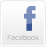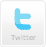HOMEMY TEACHING TOOLSFORUMABOUT USCONTACT USHome > Self-Study Quizzes > QuizGMAT Math practice - (5/36 questions - random)GMAT practice questions.  Once you complete the quiz you will see your score and explanation of the correct answer.
1.  Data Sufficiency :

Is y = 3?

(1) (y - 3)(x - 4) = 0

(2) (x - 4) = 0

2.  Which of the following inequalities have a finite range of values of "x" satisfying them?

 A) x2 + 5x + 6 > 0 B) |x + 2| > 4 C) 9x - 7 < 3x + 14 D) x2 - 4x + 3 < 0 E) (B) and (D)

3.  A wheel of a car of radius 21 cms is rotating at 600 RPM. What is the speed of the car in km/hr?

 A) 79.2 km/hr B) 47.52 km/hr C) 7.92 km/hr D) 39.6 km/hr E) 3.96 km/hr

4.  If the mean of numbers 28, x, 42, 78 and 104 is 62, then what is the mean of 128, 255, 511, 1023 and x?

 A) 395 B) 275 C) 355 D) 415 E) 365

5.  The sum of the fourth and twelfth term of an arithmetic progression is 20. What is the sum of the first 15 terms of the arithmetic progression?

 A) 300 B) 120 C) 150 D) 170 E) 270STUDENT ACTIVITIES WEBTOOLS CUSTOMER SERVICE COMPANY INFORMATION